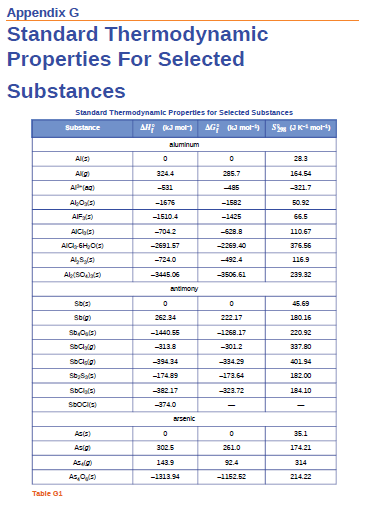# Problem: Use the standard entropy data in Appendix G to determine the change in entropy for each of the reactions listed below. All the processes occur at the standard conditions and 25 °C.(d) 2LiOH(s) + CO2(g) ⟶ Li2CO3(s) + H2O(g)

###### FREE Expert Solution

We can use the following equation to solve for ΔS˚rxn:

Note that we need to multiply each S˚ by the stoichiometric coefficient since S˚ is in J/mol • K.

83% (445 ratings)###### Problem Details

Use the standard entropy data in Appendix G to determine the change in entropy for each of the reactions listed below. All the processes occur at the standard conditions and 25 °C.

(d) 2LiOH(s) + CO2(g) ⟶ Li2CO3(s) + H2O(g)Frequently Asked Questions

What scientific concept do you need to know in order to solve this problem?

Our tutors have indicated that to solve this problem you will need to apply the Entropy concept. You can view video lessons to learn Entropy. Or if you need more Entropy practice, you can also practice Entropy practice problems.